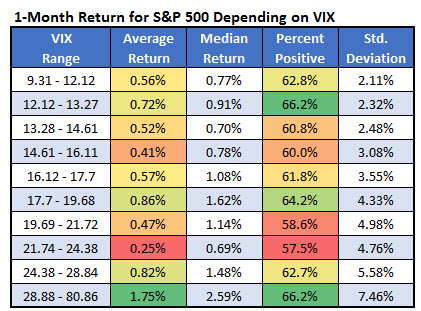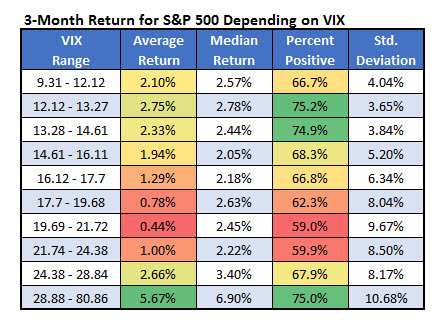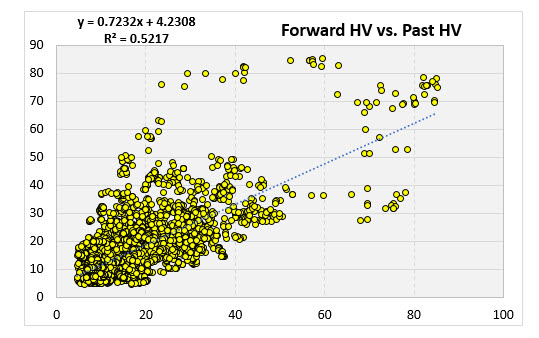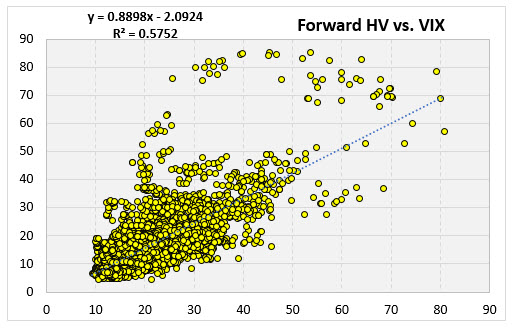# Indicator of the Week: What to Make of VIX Below 10

## Analyzing whether the CBOE Volatility Index (VIX) drop is a contrarian indicator

Senior Quantitative Analyst
May 10, 2017 at 6:30 AM

The CBOE Volatility Index (VIX) has been in the news this week. On Monday, it closed at 9.77, its lowest level since 1993, and the first close below 10 in over a decade. The VIX is often referred to as the market’s "fear gauge," since it is inclined to go up when stocks go down. The Wall Street Journal noted on their front page today that the low VIX is "signaling investor optimism about the economy and eased geopolitical concerns." Some may take this as a contrarian indicator, signaling complacency and bearish implications. This week, we will sort out what the low VIX tells us, and see if recent volatility predicts future volatility.

### Is the VIX a Contrarian Indicator?

First we will determine whether the VIX has been a contrarian indicator. I went back to 1990 (as far back as we have VIX data) and separated the VIX closing levels into 10 different brackets. I then summarized the next month's returns of the S&P 500 Index (SPX) for each of those brackets. We are currently in the first bracket below, where the VIX ranged from 9.31 to 12.12. As you go down the table, the brackets accommodate for a higher and higher VIX.

Does this show a contrarian indicator? In one sense, it does. When the VIX has been at its highest (the bottom row), the SPX has performed the best, when looking at average returns. This suggests buying stocks when the VIX is near 30. However, there is no contrarian trend toward the top side of the table. Other than the bottom bracket where VIX is at its highest, the other brackets appear random. One statistic that is predictable by the VIX is the standard deviation of returns. The lower the VIX, the smaller the standard deviation of returns. Based on the ultra-low VIX, it’s a good estimation that volatility will be muted over the next month.The table below performs the same type of analysis, except with returns over the next three months. The results are very similar. When the VIX reaches a high level, the S&P returns have outperformed over the next three months. Otherwise, the data seems rather random.### Does Recent Volatility Predict Future Volatility?

The VIX is calculated using prices of options on the SPX. Using these prices, you can calculate the implied volatility of the index. Thus, the VIX is the market’s prediction for the volatility of the S&P 500 over the next 30 days. Below we'll take a look and see if options traders are any good at predicting volatility.

What if we tried to predict future volatility simply based on recent volatility? What would be the result? Going back to 1990 on the SPX, I plotted the index’s 20-day historical volatility on the x-axis, and the volatility over the next 20 days on the y-axis. The R2 value of that trendline (shown in the upper left corner) quantifies the predictability of future volatility, given past volatility. It shows that the variation in past volatility explains 52% of the variability in future volatility.Now we will see how adept option traders are at predicting volatility. The scatter-plot below charts the VIX vs. the volatility over the next 20 days (20 trading days is roughly 30 calendar days, so the time frames match up). On this chart, you can see the R2 of the trendline is at 57.5%. In conclusion, option traders do add value to volatility predictions, but not by a determinedly wide margin. By simply using the volatility over the past 20 days, we could account for 52% of the variability in future volatility. Using only the VIX instead increases that to 57.5%.Grab your FREE Eternal Contrarian report!